SSC  >  Test: Theory of Structures

# Test: Theory of Structures

Test Description

## 20 Questions MCQ Test Mock Test Series of SSC JE Civil Engineering | Test: Theory of Structures

Test: Theory of Structures for SSC 2023 is part of Mock Test Series of SSC JE Civil Engineering preparation. The Test: Theory of Structures questions and answers have been prepared according to the SSC exam syllabus.The Test: Theory of Structures MCQs are made for SSC 2023 Exam. Find important definitions, questions, notes, meanings, examples, exercises, MCQs and online tests for Test: Theory of Structures below.
Solutions of Test: Theory of Structures questions in English are available as part of our Mock Test Series of SSC JE Civil Engineering for SSC & Test: Theory of Structures solutions in Hindi for Mock Test Series of SSC JE Civil Engineering course. Download more important topics, notes, lectures and mock test series for SSC Exam by signing up for free. Attempt Test: Theory of Structures | 20 questions in 12 minutes | Mock test for SSC preparation | Free important questions MCQ to study Mock Test Series of SSC JE Civil Engineering for SSC Exam | Download free PDF with solutions
 1 Crore+ students have signed up on EduRev. Have you?
Test: Theory of Structures - Question 1

### What are the bending moments at ends A and B of uniform fixed beam AB as shown in figure when two concentrated loads acts at 1/3 spans?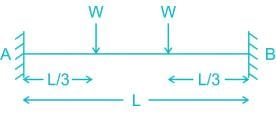Test: Theory of Structures - Question 2

### When on end of a fixed beam deflects by δ, then the bending moment at deflected end is

Test: Theory of Structures - Question 3

### If ∑H and ∑V are the algebraic sums of the forces resolved horizontally and vertically respectively, and ∑M is the algebraic sum of the moments of forces about any point, for the equilibrium of the body acted upon.

Detailed Solution for Test: Theory of Structures - Question 3

For equilibrium of any body.

(i) ∑FH = 0

(ii) ∑FV = 0

(iii) ∑MZ = 0

Test: Theory of Structures - Question 4

The force in CD of the truss shown in Fig, is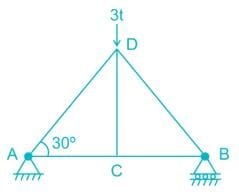Test: Theory of Structures - Question 5

A two hinge arch of span 40 m carries a point load of 62.8 KN at its crown. The Horizontal thrust in the arch is (in KN)

Test: Theory of Structures - Question 6

What is the degree of static indeterminacy of the structure shown in figure below?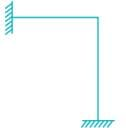Test: Theory of Structures - Question 7

If a system has more equations of equilibrium than no. of forces, then the system is:-

Detailed Solution for Test: Theory of Structures - Question 7

Let Ds = static Indeterminacy

Ds = R - r

R → no. of unknowns

r → No. of equilibrium equations available

If r > R

Ds < 0, system is partially constraintIf

Ds > 0, then system is over stiff.

Test: Theory of Structures - Question 8

Which of the following material is not used in making trusses?

Detailed Solution for Test: Theory of Structures - Question 8

Concrete is a brittle material and is unable to take tension. In Truss both tension and compression force is available, that’s why concrete is not used in making truss. While metal bars and channels are made of material which have both tensile as well as compressive strength.

Test: Theory of Structures - Question 9

What is the major difference between truss and beam?

Detailed Solution for Test: Theory of Structures - Question 9

Beam are the horizontal members which can transmit the load perpendicular to their longitudinal direction and not in axial direction while truss can transmit the load in axial direction only.

Test: Theory of Structures - Question 10

The Muller-Breslau principle in structure analysis is used for

Detailed Solution for Test: Theory of Structures - Question 10

Muller Breslau Principle: The Muller-Breslau principle states that the ILD for any stress function in a structure is represented by its deflected shape obtained by removing the restrained offered by that stress function and introducing a directly related generalised unit displacement in the direction of that stress function.

Test: Theory of Structures - Question 11

The influence line diagram for bending moment at 6 m is shown. The value of the maximum bending moment is: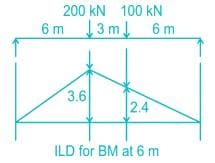Detailed Solution for Test: Theory of Structures - Question 11

The value of maximum bending moment is = 200 x 3.6 + 100 x 2.4 = 960 kN-m

Test: Theory of Structures - Question 12

The degree of static indeterminacy of the pin - jointed plane truss as shown in figure is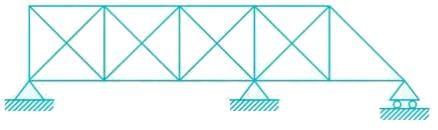Detailed Solution for Test: Theory of Structures - Question 12

Ds = m – 2j + re

m = 23

j = 11

∴ Ds = 23 – 22 + 5 = 6

Test: Theory of Structures - Question 13

Due to rising temperature in a three hinged arch, induced stress is:

Detailed Solution for Test: Theory of Structures - Question 13

Three-hinged arch is a determinate structure and rise in temperature do not produce any stress.

Test: Theory of Structures - Question 14

Which of the following equals the number of unknowns to be determined, in stiffness method of structural analysis?

Detailed Solution for Test: Theory of Structures - Question 14

Force method is useful when Ds < Dk

Displacement method is useful when Dk < D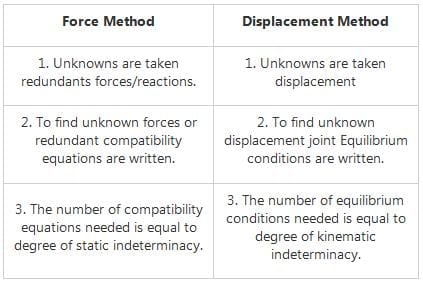Test: Theory of Structures - Question 15

The unit load method used in structural analysis is

Detailed Solution for Test: Theory of Structures - Question 15

The unit load method is extensively used in the calculation of deflection of beams, frames and trusses. Theoretically this method can be used to calculate deflections in statically determinate and indeterminate structures. However it is extensively used in evaluation of deflections of statically determinate structures only as the method requires a priori knowledge of internal stress resultants. The unit load method used in structural analysis is derived from Castigliano’s theorem.

Test: Theory of Structures - Question 16

The degree of kinematic indeterminacy of the given beam considering axial rigidity is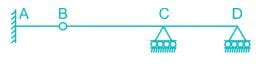Detailed Solution for Test: Theory of Structures - Question 16

Dk = 3j – re + rr

= 3 x 4 – 5 + 1 = 8

If members are considered axially rigid

Dk = 8 – 3 = 5

Test: Theory of Structures - Question 17

Consider the following statements:

The principle of superposition is not applicable when

1. The material does not obey Hooke’s law

2. The effect of temperature changes are taken into consideration

3. The structure is being analysed for the effect of support settlement

Which of these statement (s) is/are correct?

Detailed Solution for Test: Theory of Structures - Question 17

According to the principle of superposition, for a linearly elastic structure, the load effects caused by two or more loadings are the sum of the load effects caused by each loading separately. Note that the principle is limited to:

• Linear material behaviour only;

• Structures undergoing small deformations only (linear geometry).

It is not applicable when:

1. The material does not obey Hooke’s law.

2. The effect of temperature changes are taken into consideration.

3. The structure is being analysed for the effect of support settlement.

Test: Theory of Structures - Question 18

Force in the member BC of the truss shown in the given figure is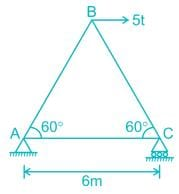Test: Theory of Structures - Question 19

Clapeyron’s theorem is associated with the analysis of

Detailed Solution for Test: Theory of Structures - Question 19
• Beams that have more than one span are defined as continuous beams. Continuous beams are very common in the bridge and building structures.
• When a beam is continuous over many supports and the moment of inertia of different spans is different, the force method of analysis becomes quite cumbersome.
• However, the force method of analysis could be further simplified for this particular case (continuous beam) by choosing the unknown bending moments at the supports as unknowns.
• One compatibility equation is written at each intermediate support of a continuous beam in terms of the loads on the adjacent span and bending moment at left, center (the support where the compatibility equation is written) and rigid supports.
• Two consecutive spans of the continuous beam are considered at one time. Since the compatibility equation is written in terms of three moments, it is known as the equation of three moments.
• In this manner, each span is treated individually as a simply supported beam with external loads and two end support moments.
• For each intermediate support, one compatibility equation is written in terms of three moments. Thus, we get as many equations as there are unknowns. Each equation will have only three unknowns.
• Clapeyron first proposed this method in 1857 and it is widely known as the Clapeyron theorem.
Test: Theory of Structures - Question 20

The given figure shows a portal frame with one end fixed and other hinged. The ratio of the fixed end moments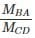due to side sway will be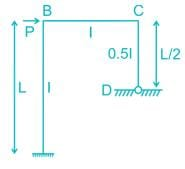Detailed Solution for Test: Theory of Structures - Question 20

Due to sway, the deflection of point B will be equal to that of point C.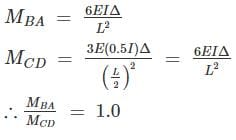## Mock Test Series of SSC JE Civil Engineering

1 videos|1 docs|64 tests
 Use Code STAYHOME200 and get INR 200 additional OFF Use Coupon Code
Information about Test: Theory of Structures Page
In this test you can find the Exam questions for Test: Theory of Structures solved & explained in the simplest way possible. Besides giving Questions and answers for Test: Theory of Structures, EduRev gives you an ample number of Online tests for practice

## Mock Test Series of SSC JE Civil Engineering

1 videos|1 docs|64 tests